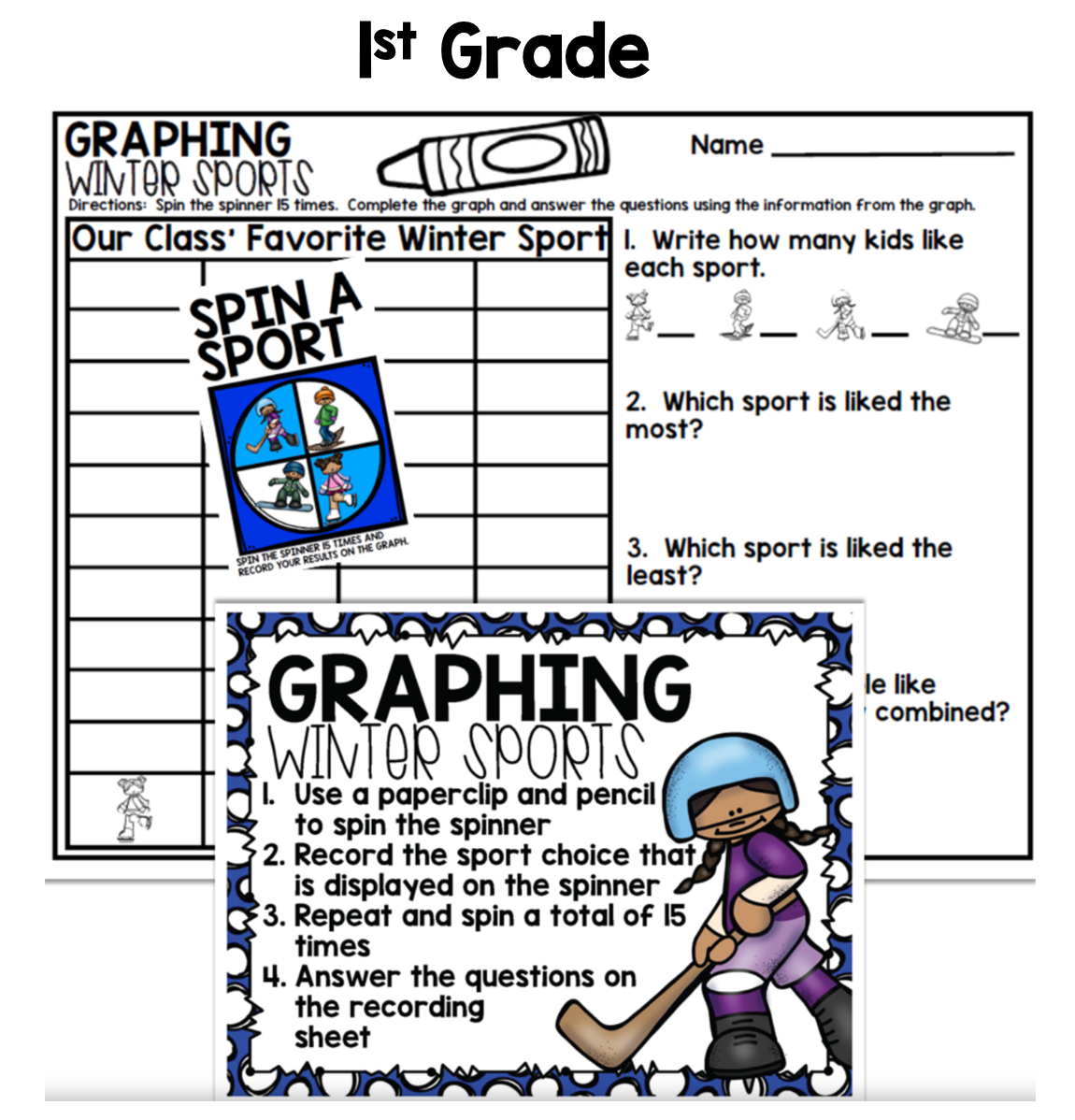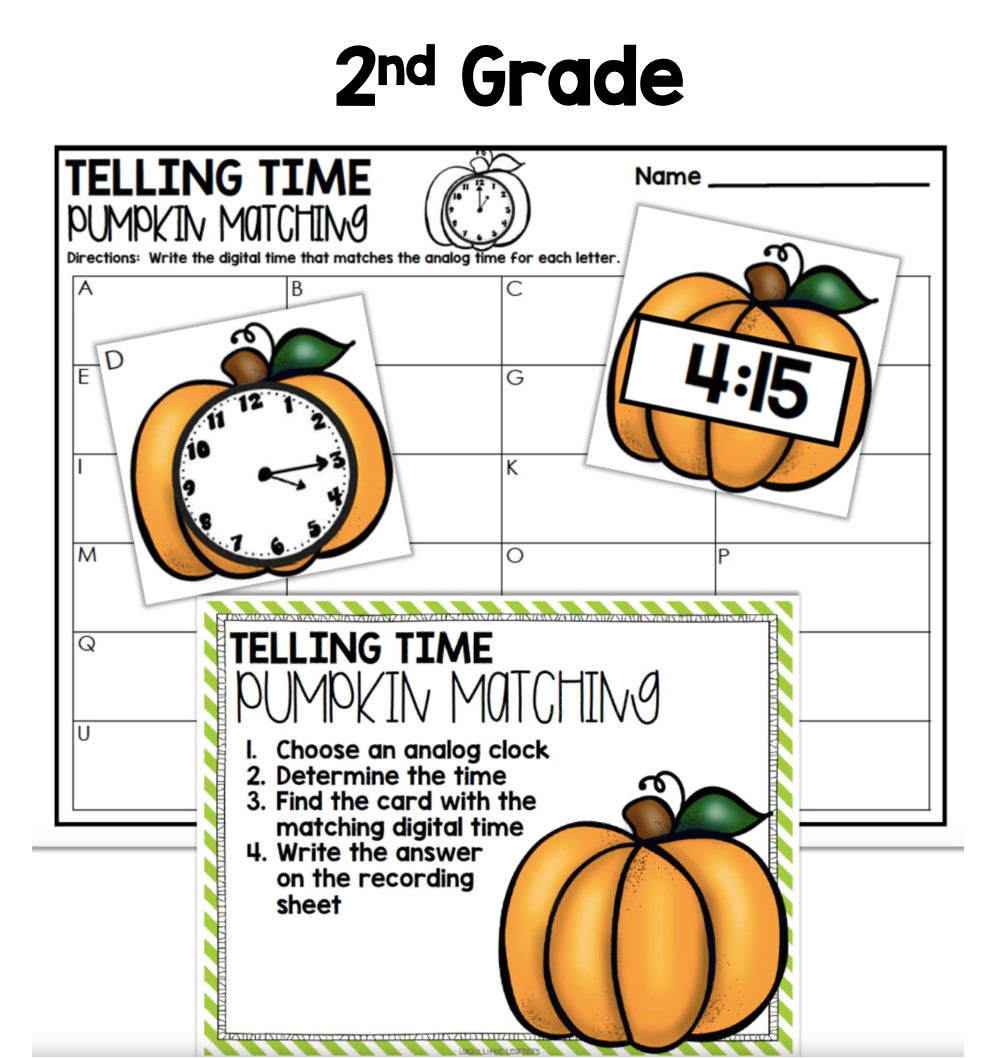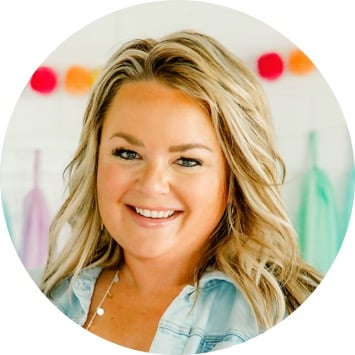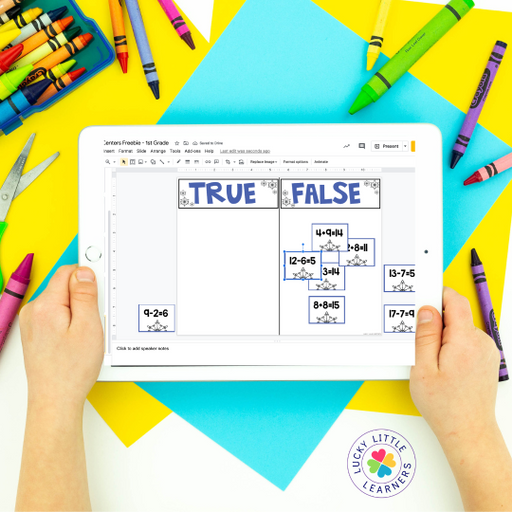Home » Blog » Math » Fall Math Centers

# Fall Math Centers

Written by: Angie Olson

It’s officially fall so it’s time to bust out those October and November Math Centers, friends! Yep, grab them out of of your file cabinet or tote and get them into your students’ hands. Let’s walk through the centers for the month! Below there are examples from both the 1st grade pack and the 2nd grade pack.

Build a turkey is a fun math center that involves the students choosing a feather. Each feather has a math fact on it. Once the student solves the math fact, they place the feather on the correct turkey with the sum of the feather.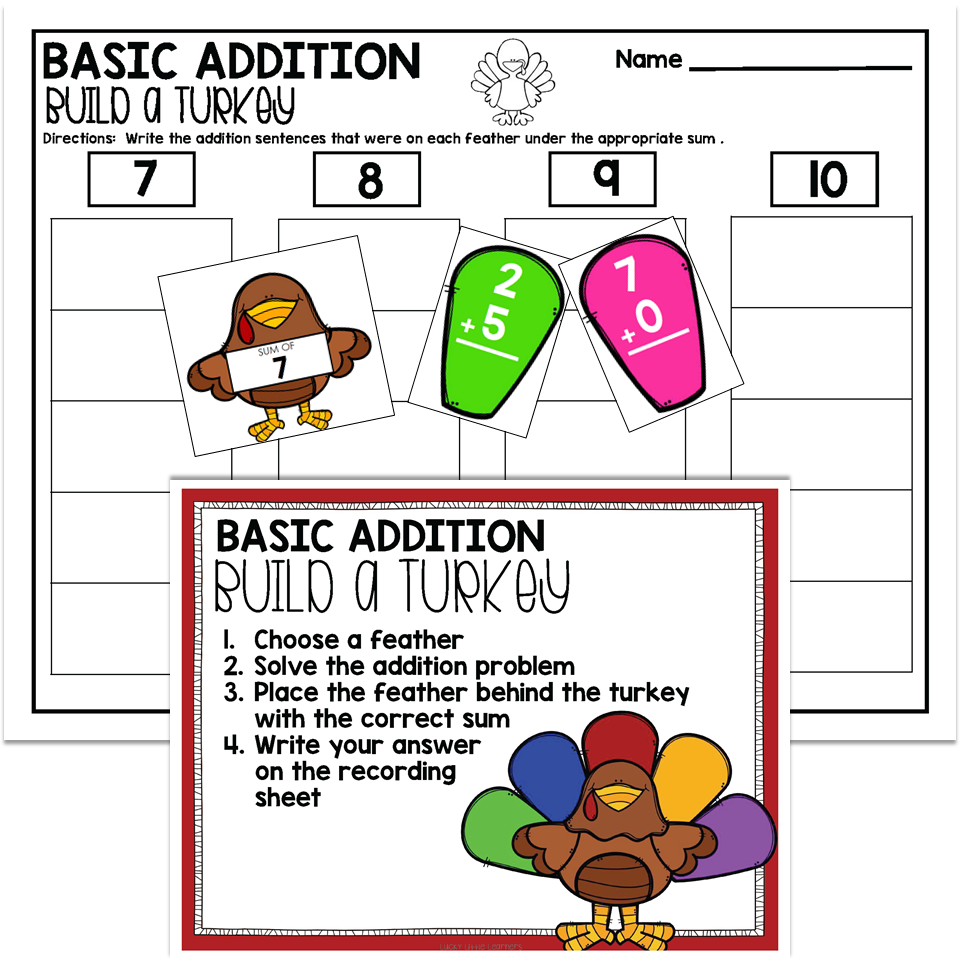## Fact Families

Students draw a house card. Each house card has three numbers. Students need to arrange the numbers to make two addition sentences and two subtraction sentences. Number cards are available or students can write the numbers using a dry erase marker.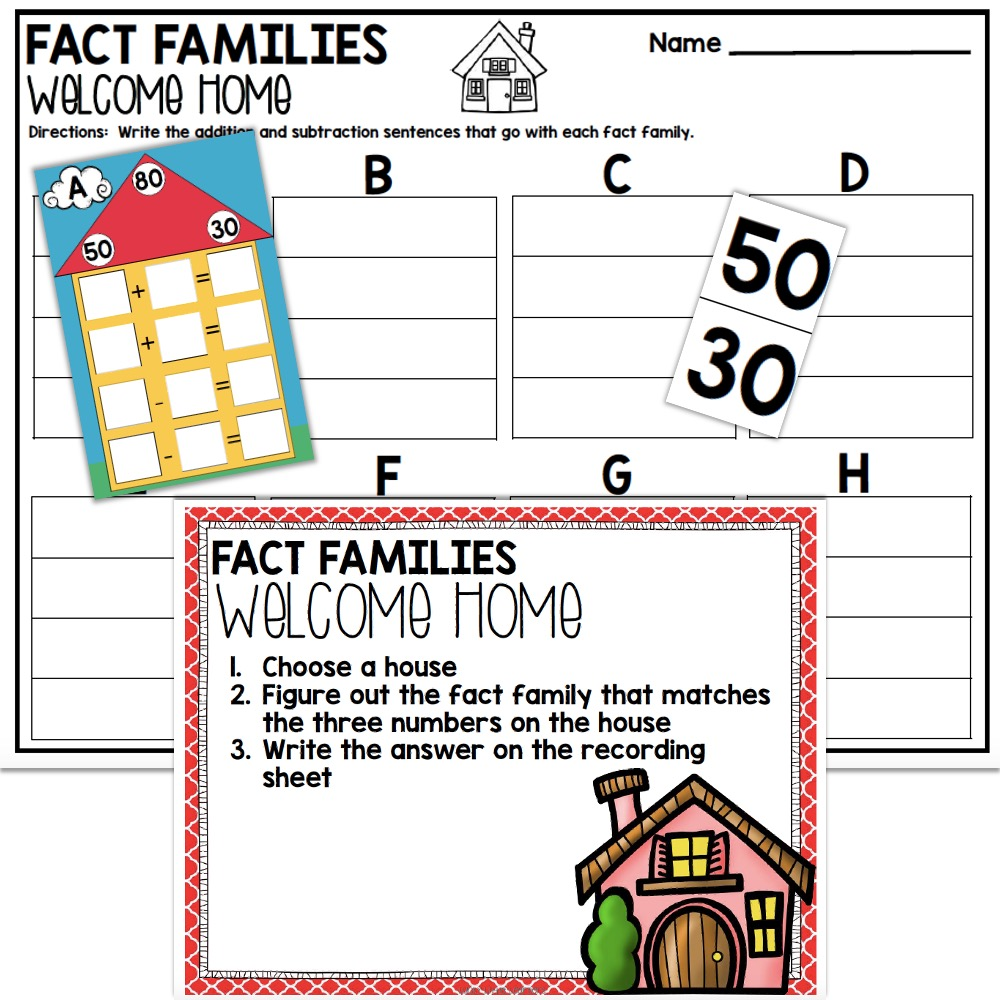Students roll three dice and place them in each roll box. Then students use counters to solve the problem. Possible counter ideas include pom poms, unifix cubes, paperclips, cereal, pennies, erasers, etc. Then the addition sentence and answer get written on the recording sheet.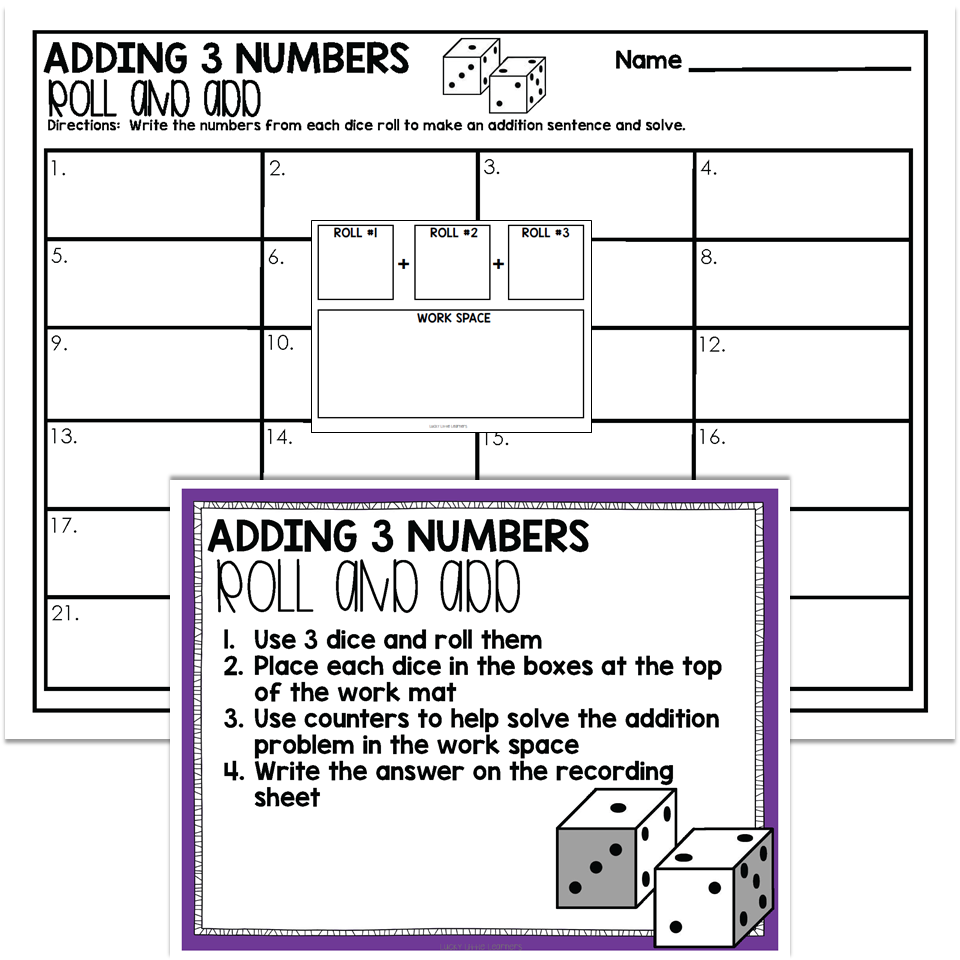## Balanced Equations

Students draw a card and add the two numbers on the left side of the card. Then students need to determine what the missing addend is for the addition sentence on the right to balance the equation.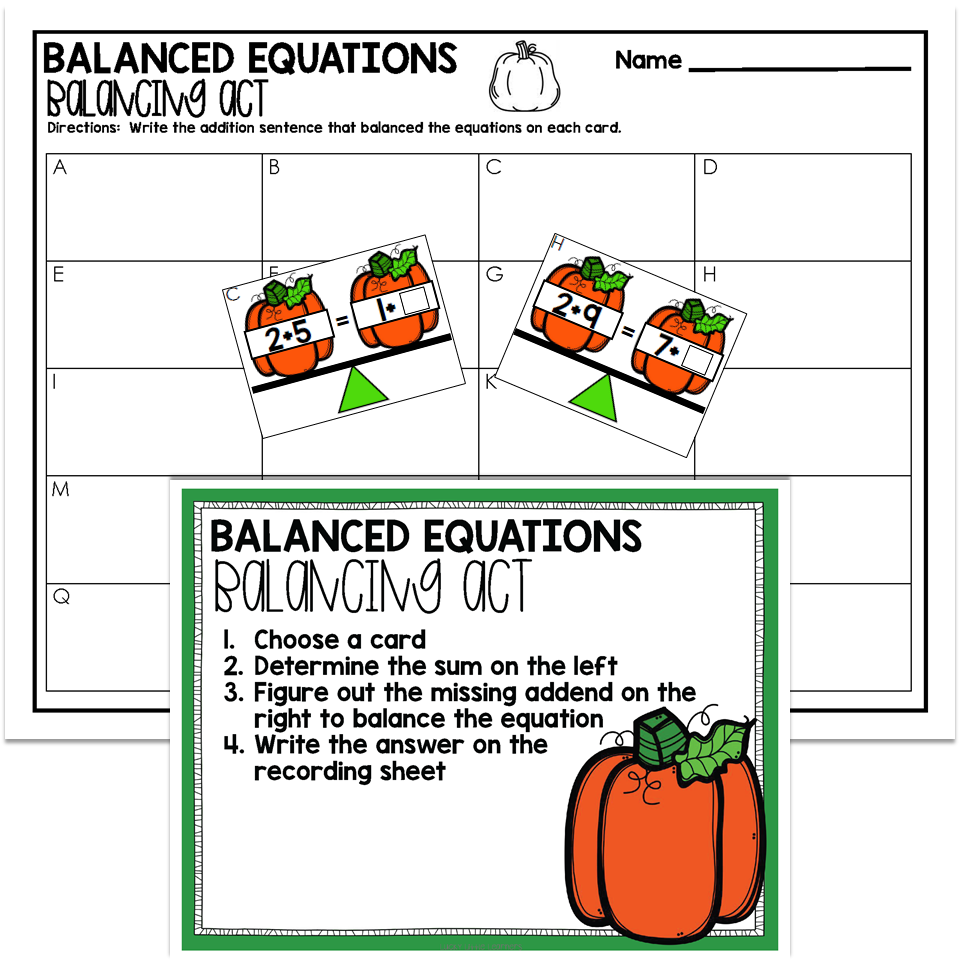## Telling Time

Have your students practice telling time both with the digital clock and the analog clock with this center. Students draw a clock card and then find another clock card that displays the same time.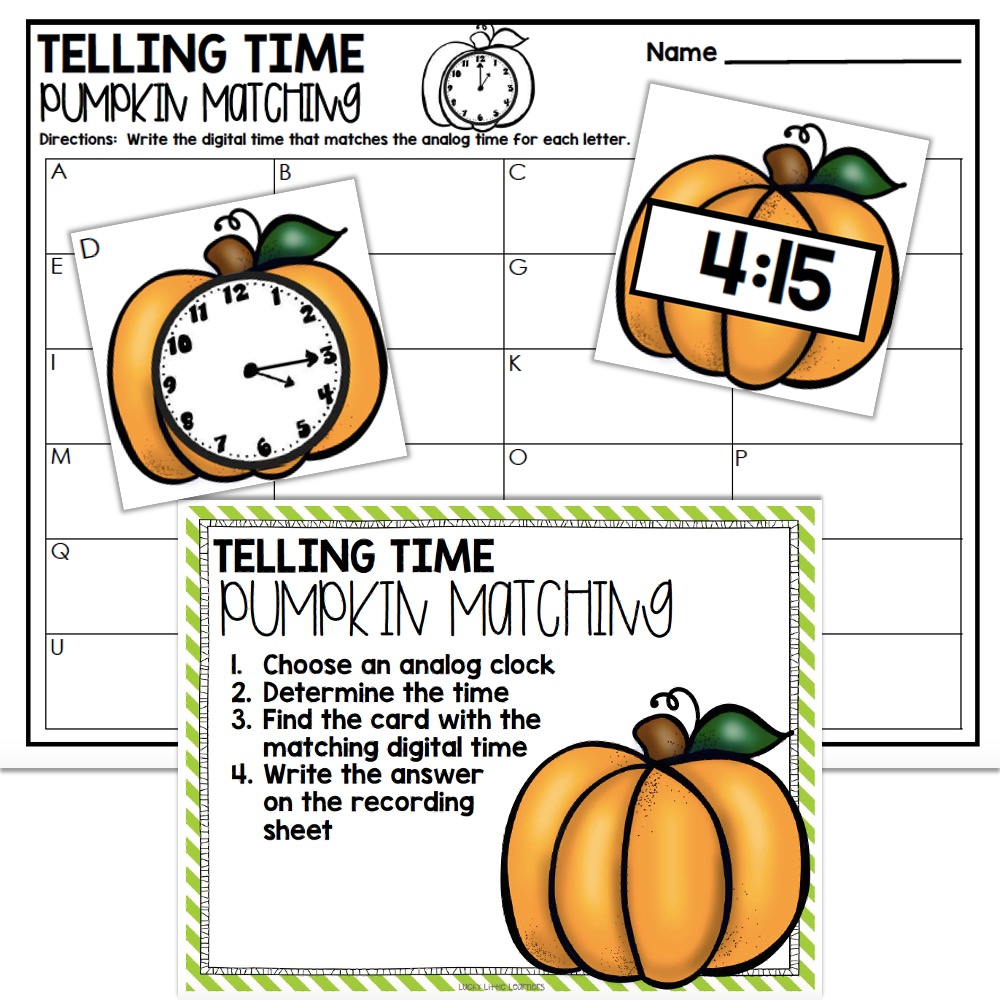## Greater, Less, and Equal

Give students 4 dice and have them roll to form two 2-digit numbers. Students then determine whether to use a greater than, less than, or equal to symbol between the two numbers to make a true number comparison.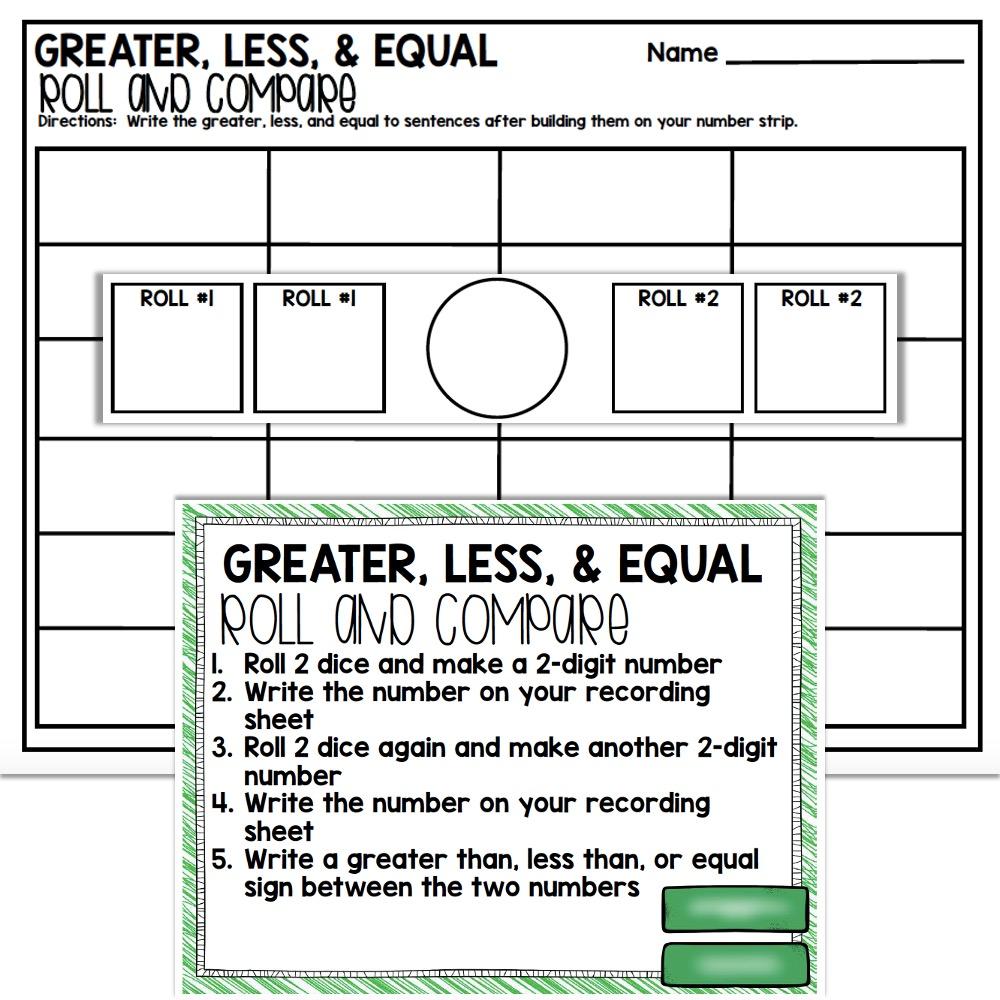## Subtraction

Students draw a puzzle card with a subtraction problem. Students will use the tools they need to use to solve the subtraction problem. Then students will find the answer to the subtraction problem and place the answer card underneath the problem to complete the puzzle.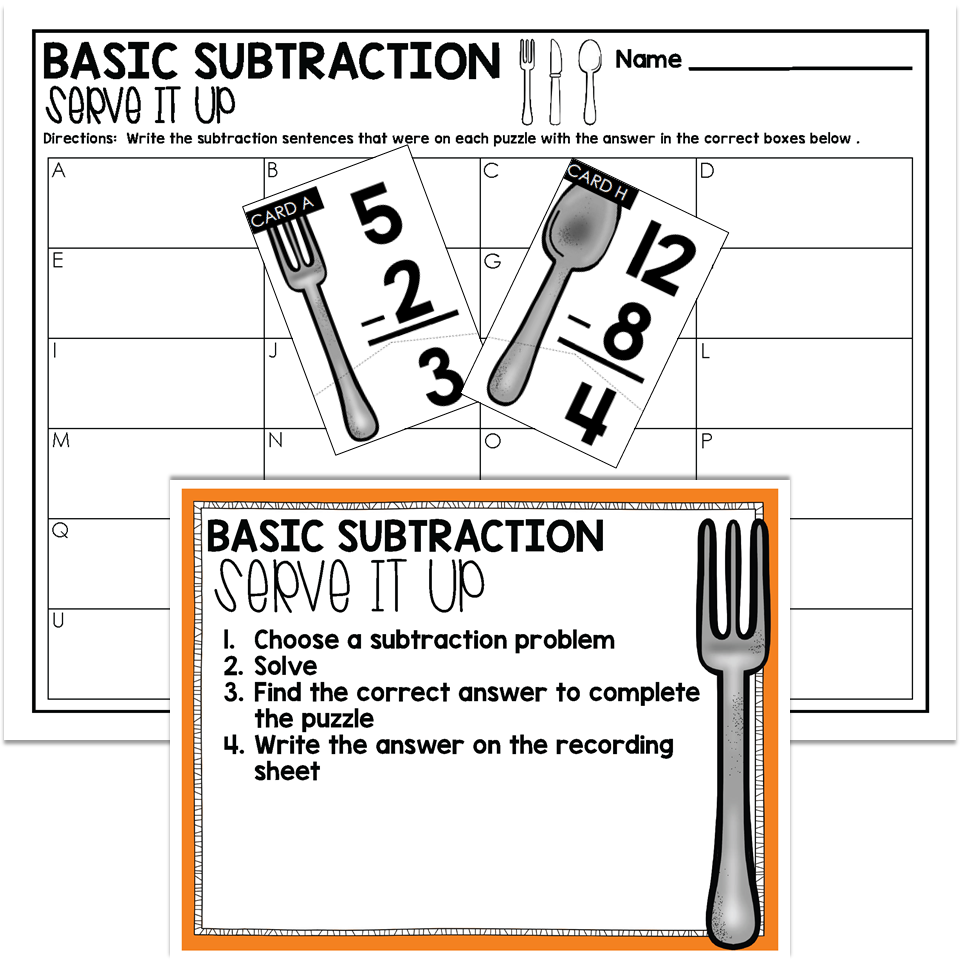## Money Identifying & Counting

Students draw a card and find the total number of coins that are found on the card. Then students add up the coin values to find a sum. The total value of all the coins get written on the recording sheet.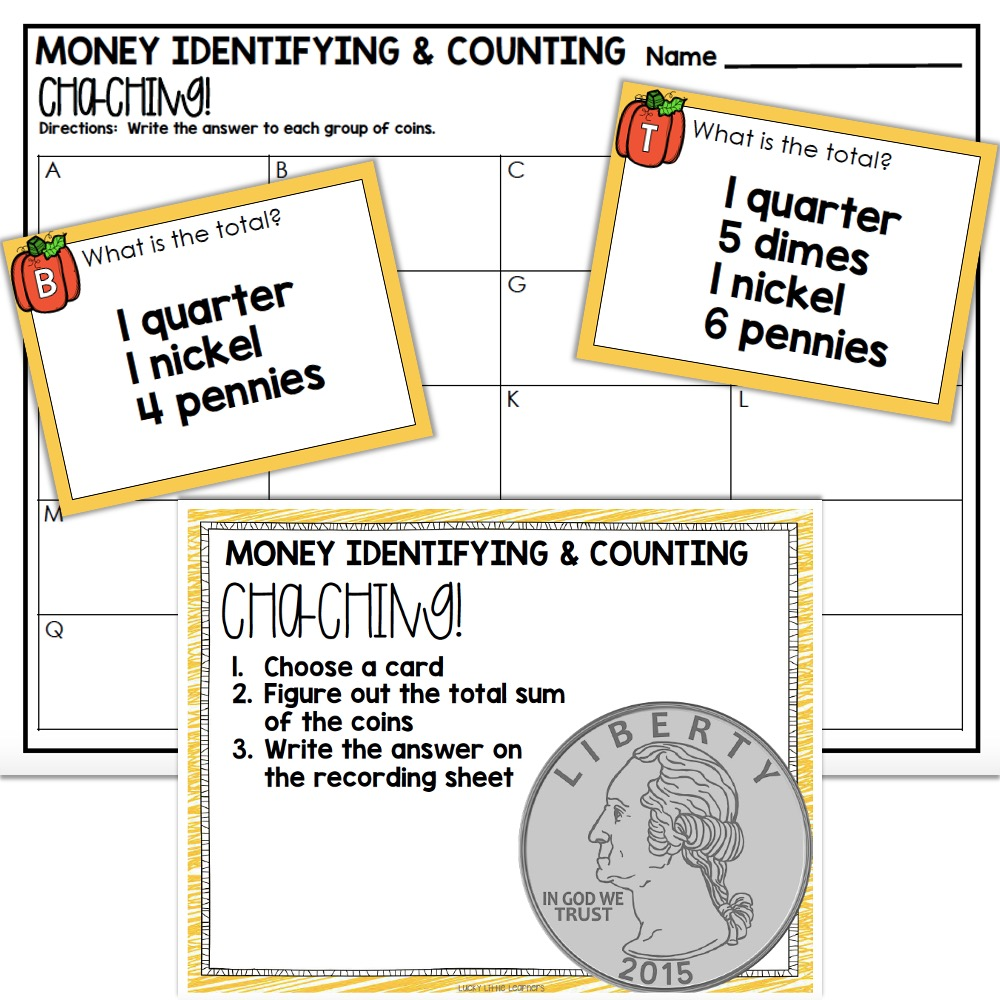## Ordinal Numbers

Students choose a puzzle piece that shows fingers first. Then the students find the matching number word and ordinal number pieces to complete the puzzle.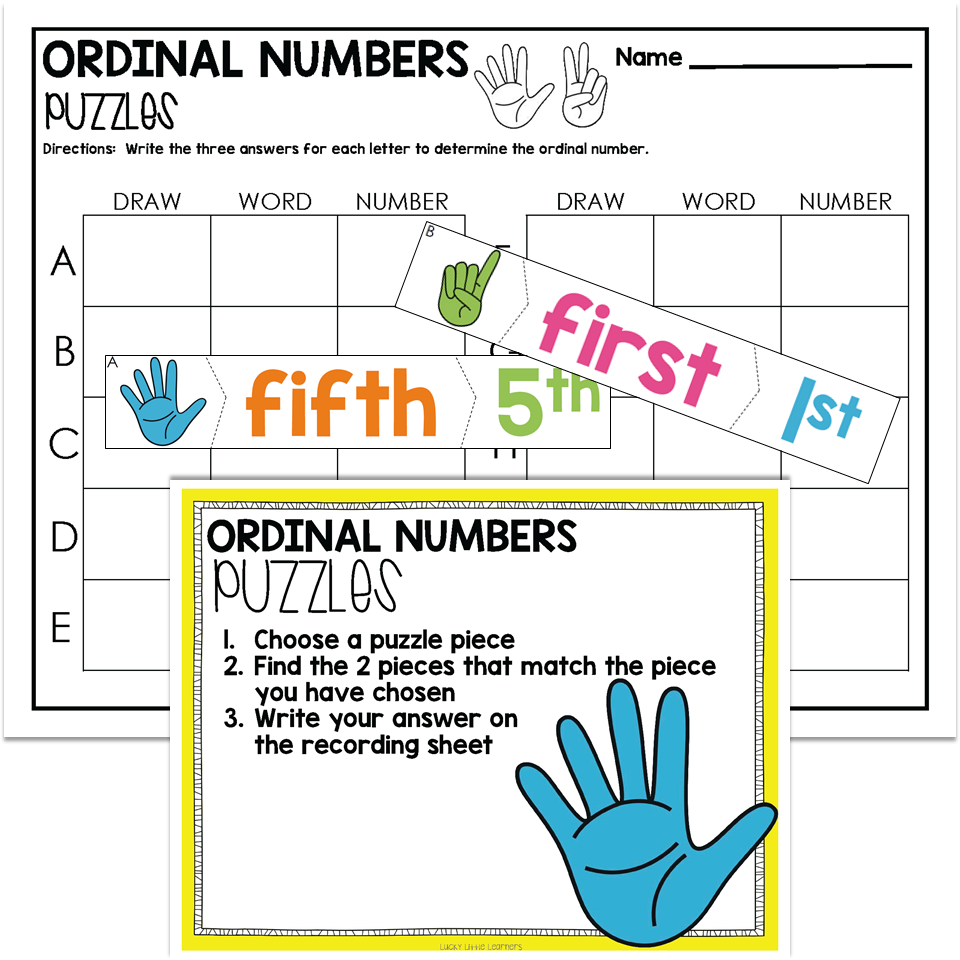## Skip Counting

Students draw a number sequence and say the number sequence aloud. Then students determine what the missing number in the sequence is and find that number card to place on the number sequence.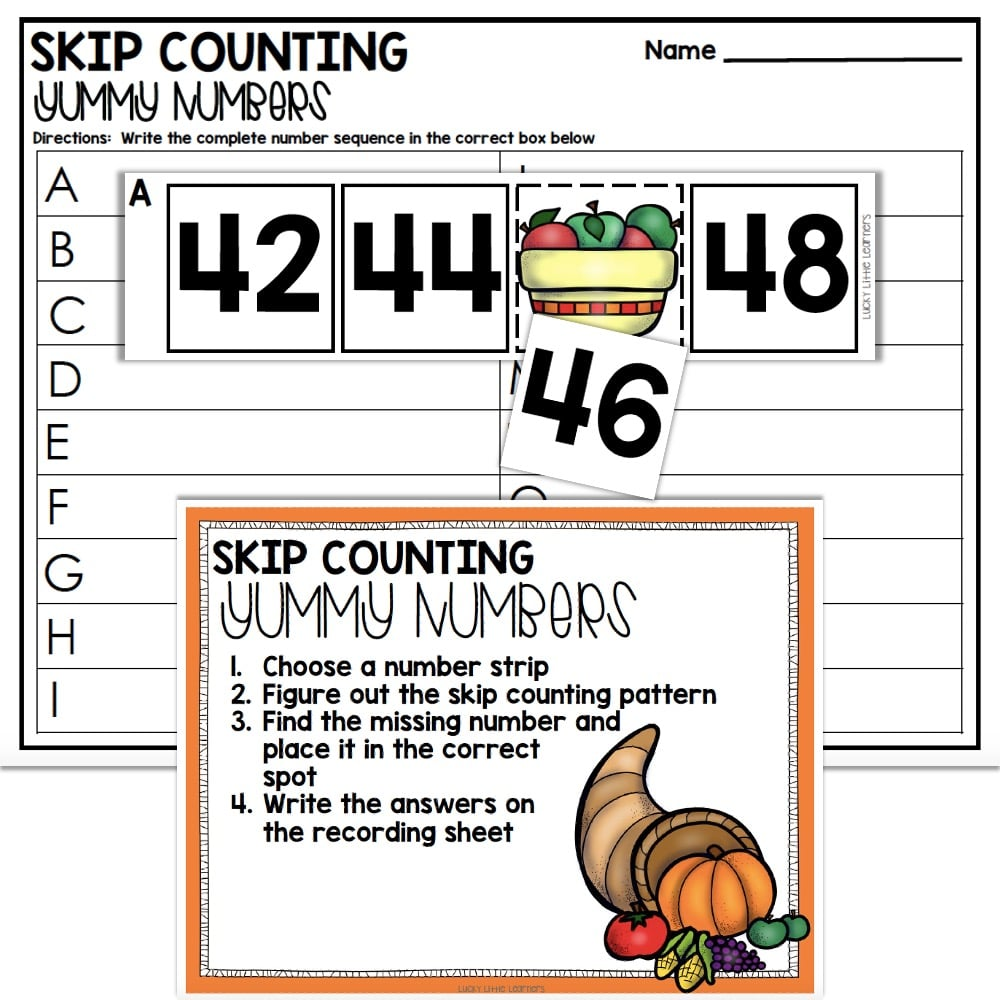## Place Value

Practice place value skills with Build the Number. Students draw a card and then use their place value mat and place value blocks to show the number of hundreds, tens, and ones that are needed to create the number.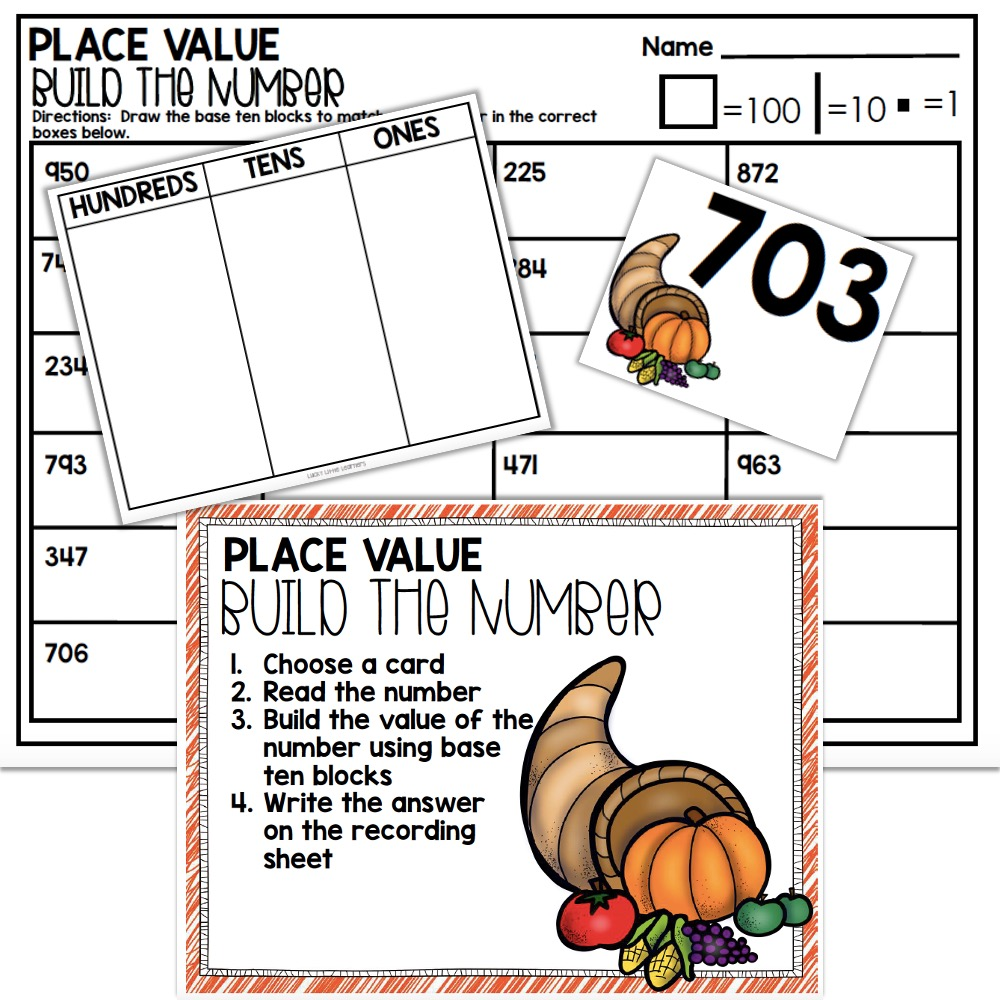## 1st and 2nd Grade Math Centers

Grab your math centers today buy purchasing the full year bundle. The full year bundles are available for both 1st grade and 2nd grade. Each bundle includes 120 math centers that are both full color and black and white. Every math center comes with a recording sheet for accountability along with answer keys.